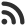Feed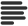Articles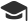Tutorials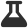CodingLab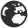CommunityLeaderboardFounder & Programmer at HackersFriend Updated July 16, 2019, 3:24 p.m. ⋅ 270 views

# Count duplicates in a given linked list

Problem

You are given a linked list. Your task is to find out all the duplicates in it and print the count of nodes which has at least 1 duplicate.  Have a look at example.

Example

Input 1

``5 -> 9 -> 5 -> 1 -> 9 -> NULL``

Output 1

``2``

Explanation

Here we can see 5 has a duplicate and 9 has a duplicate in linked list. So, in total we have 2 nodes which has duplicates in linked list, so our answer would be 2.

Input 2

`` 5 -> 3 -> 8 -> 3 -> 1 -> NULL``

Output 2

``1``

Explanation

Here, we can see 3 is the only element which has a duplicate in linked list. So, our answer would be 1.

Solution

Naive apporach

The simplest way to solve this problem is to traverse the whole linked list and for each element we'll traverse the rest of the linked list and see if there is any duplicate, if duplicate is found, we'll increment the duplicate couter.

Here is implementation of this approach.

``````#include <bits/stdc++.h>
using namespace std;

// node
struct Node {
int data;
Node* next;
};

// Function to insert a node at the beginning
{
Node* temp = new Node();
temp->data = item;
}

// Function to count the number of
// duplicate nodes in the linked list
{
int count = 0;

// Starting from the next node
while (ptr != NULL) {

// If some duplicate node is found
count++;
break;
}
ptr = ptr->next;
}

}

// Return the count of duplicate nodes
return count;
}

// Main function
int main()
{

return 0;
}
``````
``2``

Time complexity of this approach would be O(n2)

Efficient approach

For problems like this, in which we have to find duplicates in a list, we can use hashing.

We will build a hash table and store the data into hash and for each element we'll check if that element exists in hash, if it does, we'll increment duplicate counter. Finally, duplicate counter will be our answer which we'll return from function.

Here is implementation of this approach.

``````#include <bits/stdc++.h>
using namespace std;

// node
struct Node {
int data;
Node* next;
};

// Function to insert a node at the beginning
{
Node* temp = new Node();
temp->data = item;
}

// Function to count the number of
// duplicate nodes in the linked list
{
return 0;;

// Create a hash table insert head
unordered_set<int> s;

// Traverse through remaining nodes
int count = 0;
for (Node *curr=head->next; curr != NULL; curr=curr->next) {
if (s.find(curr->data) != s.end())
count++;

s.insert(curr->data);
}

// Return the count of duplicate nodes
return count;
}

// Main Function
int main()
{

return 0;
}
``````
``2``

Time complexity of this approach would be O(n).

 arrow_upward UpvoteComment arrow_downward downvote

Events

Nov. 28, 2018, 5:30 p.m.

Python from zero to hero

place Delhi

Aug. 13, 2018, 5:30 p.m.

Python from zero to hero

place Bangalore ( HackersFriend office BTM Layout)